Theory and Modern Applications

# Robust stability and stabilization of uncertain switched discrete-time systems

## Abstract

This paper is concerned with the robust stability and stabilization for a class of switched discrete-time systems with state parameter uncertainty. Firstly, a new matrix inequality considering uncertainties is introduced and proved. By means of it, a novel sufficient condition for robust stability and stabilization of a class of uncertain switched discrete-time systems is presented. Furthermore, based on the result obtained, the switching law is designed and has been performed well, and some sufficient conditions of robust stability and stabilization have been derived for the uncertain switched discrete-time systems using the Lyapunov stability theorem, block matrix method, and inequality technology. Finally, some examples are exploited to illustrate the effectiveness of the proposed schemes.

## 1 Introduction

A switched system is a hybrid dynamical system consisting of a finite number of subsystems and a logical rule that manages switching between these subsystems. Switched systems have drawn a great deal of attention in recent years; see  and references therein. The motivation for studying switched systems comes partly from the fact that switched systems and switched multicontroller systems have numerous applications in control of mechanical systems, process control, automotive industry, power systems, aircraft and traffic control, and many other fields. An important qualitative property of switched system is stability . The challenge of analyzing the stability of switched system lies partly in the fact that, even if the individual systems are stable, the switched system might be unstable. Using a common quadratic Lyapunov function on all subsystems, the quadratic Lyapunov stability facilitates the analysis and synthesis of switched systems. However, the obtained results within this framework have been recognized to be conservative. In , various algorithms both for stability and performance analysis of discrete-time piece-wise affine systems were presented. Different classes of Lyapunov functions were considered, and how to compute them through linear matrix inequalities was also shown. Moreover, the tradeoff between the degree of conservativeness and computational requirements was discussed. The problem of stability analysis and control synthesis of switched systems in the discrete-time domain was addressed in . The approach followed in  looked at the existence of a switched quadratic Lyapunov function to check asymptotic stability of the switched system under consideration. Two different linear matrix inequality-based conditions allow to check the existence of such a Lyapunov function. These two conditions have been proved to be equivalent for stability analysis.

There are many methodologies and approaches developed in the switched systems theory: approaches of looking for an appropriate switching strategy to stabilize the system , dwell-time and average dwell-time approaches for stability analysis and stabilization problems , approaches of studying stability and control problems under a specific class of switching laws , or under arbitrary switching sequences [6, 9]. Reference  investigated the quadratic stability and linear state feedback and output feedback stabilization of switched delayed linear dynamic systems with, in general, a finite number of noncommensurate constant internal point delays. The results were obtained based on Lyapunov stability analysis via appropriate Krasovskii-Lyapunov functionals, and the related stability study was performed to obtain both delay-independent and delay-dependent results. The problem of fault estimation for a class of switched nonlinear systems of neutral type was considered in . Sufficient delay-dependent existence conditions of the ${H}_{\mathrm{\infty }}$ fault estimator were given in terms of certain matrix inequalities based on the average dwell-time approach. The problem of robust reliable control for a class of uncertain switched neutral systems under asynchronous switching was investigated in . A state feedback controller was proposed to guarantee exponential stability and reliability for switched neutral systems, and the dwelltime approach was utilized for the stability analysis and controller design. The exponential stability for a class of nonlinear hybrid time-delay systems was addressed in . The delay-dependent stability conditions were presented in terms of the solution of algebraic Riccati equations, which allows computing simultaneously the two bounds that characterize the stability rate of the solution.

On another research front line, it has been recognized that parameter uncertainties, which often occur in many physical processes, are main sources of instability and poor performance. Therefore, much attention has been devoted to the study of various systems with uncertainties, and a great number of useful results have been reported in the literature on the issues of robust stability, robust ${H}_{\mathrm{\infty }}$ control, robust ${H}_{\mathrm{\infty }}$ filtering, and so on, by considering different classes of parameter uncertainties [12, 14].

Recently, some stability condition and stabilization approaches have been proposed for the switched discrete-time system [15, 18]. In , the quadratic stabilization of discrete-time switched linear systems was studied, and quadratic stabilization of switched systems with norm bounded time varying uncertainties was investigated. In , the stability property for the switched systems which were composed of a continuous-time LTI subsystem and a discrete time LTI subsystem was studied. There existed a switched quadratic Lyapunov function to check asymptotic stability of the switched discrete-time system in .

The objective of this paper is to present novel approaches for the asymptotical stability and stabilization of switched discrete-time system with parametric uncertainties. The parameter uncertainties are time-varying but norm-bounded. Firstly, a new inequality is given. Using the new result, a new sufficient condition for robust stability and stabilization of a class of uncertain switched discrete-time systems is proposed. Furthermore, using the block matrix method, inequality technology, and the Lyapunov stability theorem, some sufficient conditions for robust stability and stabilization have been presented for the uncertain switched discrete-time systems, and the switched law design has been performed. Comparing with [22, 23], the uncertainty in system was not considered in [22, 23], but we consider the uncertainty in systems and the design switching law is simple and easy for application.

The rest of this paper is organized as follows. The problem is formulated in Section 2. Section 3 deals with robust stability and stabilization criteria for a class of discrete-time switched system with uncertainty. Numerical examples are provided to illustrate the theoretical results in Section 4, and the conclusions are drawn in Section 5.

## 2 Preliminaries

The following notations will be used throughout this paper. ${R}^{+}$ denotes the set of all real nonnegative numbers; ${R}^{n}$ denotes the n-dimensional space with the scalar product of two vectors $〈x,y〉$ or ${x}^{T}y$; ${R}^{n×r}$ denotes the space of all matrices of $\left(n×r\right)$-dimension. ${A}^{T}$ denotes the transpose of A; a matrix A is symmetric if $A={A}^{T}$.

Matrix A is semipositive definite ($A\ge 0$) if $〈Ax,x〉\ge 0$, for all $x\in {R}^{n}$; A is positive definite ($A>0$) if $〈Ax,x〉>0$ for all $x\ne 0$; $A\ge B$ means $A-B\ge 0$. $\lambda \left(A\right)$ denotes the set of all eigenvalues of A; ${\lambda }_{min}\left(A\right)=min\left\{Re\lambda :\lambda \in \lambda \left(A\right)\right\}$.

Consider uncertain discrete systems with interval time-varying delay of the form

$\begin{array}{r}x\left(k+1\right)=\left({A}_{\gamma }+\mathrm{\Delta }{A}_{\gamma }\left(k\right)\right)x\left(k\right)+\left({B}_{\gamma }+\mathrm{\Delta }{B}_{\gamma }\left(k\right)\right)x\left(k-d\left(k\right)\right),\phantom{\rule{1em}{0ex}}k=0,1,2,\dots ,\\ x\left(k\right)={v}_{k},\phantom{\rule{1em}{0ex}}k=-{d}_{2},-{d}_{2}+1,\dots ,0,\end{array}$
(2.1)

where $x\left(k\right)\in {R}^{n}$ is the state, $\gamma \left(\cdot \right):{R}^{n}\to \mathcal{N}:=\left\{1,2,\dots ,N\right\}$ is the switching rule, which is a function depending on the state at each time and will be designed. A switching function is a rule which determines a switching sequence for a given switching system. Moreover, $\gamma \left(x\left(k\right)\right)=i$ implies that the system realization is chosen as the i th system, $i=1,2,\dots ,N$. It is seen that the system (2.1) can be viewed as an autonomous switched system in which the effective subsystem changes when the state $x\left(k\right)$ hits predefined boundaries. ${A}_{i}$, ${B}_{i}$, $i=1,2,\dots ,N$ are given constant matrices and the time-varying uncertain matrices $\mathrm{\Delta }{A}_{i}\left(k\right)$ and $\mathrm{\Delta }{B}_{i}\left(k\right)$ are defined by

$\mathrm{\Delta }{A}_{i}\left(k\right)={E}_{ia}{F}_{ia}\left(k\right){H}_{ia},\mathrm{\Delta }{B}_{i}\left(k\right)={E}_{ib}{F}_{ib}\left(k\right){H}_{ib},$
(2.2)

where ${E}_{ia}$, ${E}_{ib}$, ${H}_{ia}$, ${H}_{ib}$ are known constant real matrices with appropriate dimensions.

${F}_{ia}\left(k\right)$, ${F}_{ib}\left(k\right)$ are unknown uncertain matrices satisfying

${F}_{ia}^{T}\left(k\right){F}_{ia}\left(k\right)\le I,\phantom{\rule{2em}{0ex}}{F}_{ib}^{T}\left(k\right){F}_{ib}\left(k\right)\le I,\phantom{\rule{1em}{0ex}}k=0,1,2,\dots .$
(2.3)

The time-varying function $d\left(k\right)$ satisfies the following condition:

$0<{d}_{1}\le d\left(k\right)\le {d}_{2},\phantom{\rule{1em}{0ex}}\mathrm{\forall }k=0,1,2,\dots .$

Remark 2.1 It is worth noting that the time delay is a time-varying function belonging to a given interval, in which the lower bound of delay is not restricted to zero.

Definition 2.1 The uncertain switched system (2.1) is robustly stable if there exists a switching function $\gamma \left(\cdot \right)$ such that the zero solution of the uncertain switched system is asymptotically stable for all uncertainties which satisfy (2.2) and (2.3).

Definition 2.2 The system of matrices $\left\{{J}_{i}\right\}$, $i=1,2,\dots ,N$, is said to be strictly complete if for every $x\in {R}^{n}\setminus \left\{0\right\}$ there is $i\in \left\{1,2,\dots ,N\right\}$ such that ${x}^{T}{J}_{i}x<0$.

It is easy to see that the system $\left\{{J}_{i}\right\}$ is strictly complete if and only if

$\bigcup _{i=1}^{N}{\alpha }_{i}={R}^{n}\setminus \left\{0\right\},$

where

${\alpha }_{i}=\left\{x\in {R}^{n}:{x}^{T}{J}_{i}x<0\right\},\phantom{\rule{1em}{0ex}}i=1,2,\dots ,N.$

Proposition 2.1 ()

The system$\left\{{J}_{i}\right\}$, $i=1,2,\dots ,N$, is strictly complete if there exist${\delta }_{i}\ge 0$, $i=1,2,\dots ,N$, ${\sum }_{i=1}^{N}{\delta }_{i}>0$such that

$\sum _{i=1}^{N}{\delta }_{i}{J}_{i}<0.$

If$N=2$then the above condition is also necessary for the strict completeness.

Proposition 2.2 (Cauchy inequality)

For any symmetric positive definite matrix $N\in {M}^{n×n}$ and $a,b\in {R}^{n}$ we have

$\underline{+}{a}^{T}b\le {a}^{T}Na+{b}^{T}{N}^{-1}b.$

Proposition 2.3 ()

Let E, H and F be any constant matrices of appropriate dimensions and${F}^{T}F\le I$. For any$ϵ>0$, we have

$EFH+{H}^{T}{F}^{T}{E}^{T}\le ϵE{E}^{T}+{ϵ}^{-1}{H}^{T}H.$

## 3 Main results

### 3.1 Stability

Let us set

${W}_{i}\left({S}_{1},{S}_{2},P,Q\right)=\left[\begin{array}{ccc}{W}_{i11}& {W}_{i12}& {W}_{i13}\\ \ast & {W}_{i22}& {W}_{i23}\\ \ast & \ast & {W}_{i33}\end{array}\right],$

where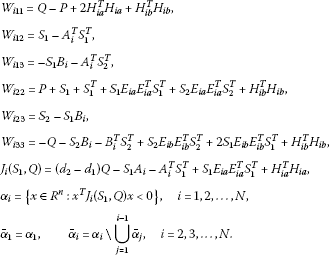(3.1)

The main result of this paper is summarized in the following theorem.

Theorem 3.1 The uncertain switched system (2.1) is robustly stable if there exist symmetric positive definite matrices$P>0$, $Q>0$and matrices${S}_{1}$, ${S}_{2}$satisfying the following conditions

1. (i)

$\mathrm{\exists }{\delta }_{i}\ge 0$, $i=1,2,\dots ,N$, ${\sum }_{i=1}^{N}{\delta }_{i}>0:{\sum }_{i=1}^{N}{\delta }_{i}{J}_{i}\left({S}_{1},Q\right)<0$,

2. (ii)

${W}_{i}\left({S}_{1},{S}_{2},P,Q\right)<0$, $i=1,2,\dots ,N$.

The switching rule is chosen as$\gamma \left(x\left(k\right)\right)=i$, whenever$x\left(k\right)\in {\overline{\alpha }}_{i}$.

Proof Consider the following Lyapunov-Krasovskii functional for any i th system (2.1)

$V\left(k\right)={V}_{1}\left(k\right)+{V}_{2}\left(k\right)+{V}_{3}\left(k\right),$

where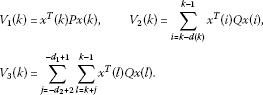We can verify that

${\lambda }_{1}{\parallel x\left(k\right)\parallel }^{2}\le V\left(k\right).$
(3.2)

Let us set $\xi \left(k\right)={\left[{x}^{T}\left(k\right){x}^{T}\left(k+1\right){x}^{T}\left(k-d\left(k\right)\right)\right]}^{T}$, and

$H=\left(\begin{array}{ccc}0& 0& 0\\ 0& P& 0\\ 0& 0& 0\end{array}\right),\phantom{\rule{2em}{0ex}}G=\left(\begin{array}{ccc}P& 0& 0\\ I& I& 0\\ 0& 0& I\end{array}\right).$

Then, the difference of ${V}_{1}\left(k\right)$ along the solution of the system is given by

$\begin{array}{rcl}\mathrm{\Delta }{V}_{1}\left(k\right)& =& {x}^{T}\left(k+1\right)Px\left(k+1\right)-{x}^{T}\left(k\right)Px\left(k\right)\\ =& {\xi }^{T}\left(k\right)H\xi \left(k\right)-2{\xi }^{T}\left(k\right){G}^{T}\left(\begin{array}{c}0.5x\left(k\right)\\ 0\\ 0\end{array}\right),\end{array}$
(3.3)

because of

${\xi }^{T}\left(k\right)H\xi \left(k\right)=x\left(k+1\right)Px\left(k+1\right).$

Using the expression of system (2.1)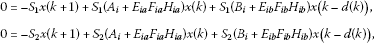we have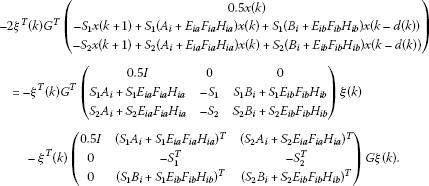Therefore, from (3.3), it follows that

$\begin{array}{rcl}\mathrm{\Delta }{V}_{1}\left(k\right)& =& {x}^{T}\left(k\right)\left[-P-{S}_{1}\left({A}_{i}+{E}_{ia}{F}_{ia}{H}_{ia}\right)-{\left({A}_{i}+{E}_{ia}{F}_{ia}{H}_{ia}\right)}^{T}{S}_{1}^{T}\right]x\left(k\right)\\ +2{x}^{T}\left(k\right)\left[{S}_{1}-{A}_{i}^{T}{S}_{1}^{T}-{\left({E}_{ia}{F}_{ia}{H}_{ia}\right)}^{T}{S}_{1}^{T}\right]x\left(k+1\right)\\ +2{x}^{T}\left(k\right)\left[-{S}_{1}{B}_{i}-{S}_{1}\left({E}_{ib}{F}_{ib}{H}_{ib}\right)-{A}_{i}^{T}{S}_{2}^{T}-{\left({E}_{ia}{F}_{ia}{H}_{ia}\right)}^{T}{S}_{2}^{T}\right]x\left(k-h\left(k\right)\right)\\ +x\left(k+1\right)\left[P+{S}_{1}+{S}_{1}^{T}\right]x\left(k+1\right)\\ +2x\left(k+1\right)\left[{S}_{2}-{S}_{1}{B}_{i}-{S}_{1}\left({E}_{ib}{F}_{ib}{H}_{ib}\right)\right]x\left(k-h\left(k\right)\right)\\ +x\left(k-h\left(k\right)\right)\left[-{S}_{2}\left({B}_{i}+{E}_{ib}{F}_{ib}{H}_{ib}\right)-{\left({B}_{i}+{E}_{ib}{F}_{ib}{H}_{ib}\right)}^{T}{S}_{2}^{T}\right]x\left(k-h\left(k\right)\right).\end{array}$

Applying Proposition 2.2, Proposition 2.3 and condition (2.2), the following estimations hold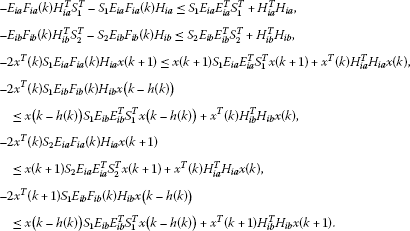Therefore, we have

$\begin{array}{rcl}\mathrm{\Delta }{V}_{1}\left(k\right)& \le & {x}^{T}\left(k\right)\left[-P-{S}_{1}{A}_{i}-{A}_{i}^{T}{S}_{1}^{T}+{S}_{1}{E}_{ia}{E}_{ia}^{T}{S}_{1}^{T}+3{H}_{ia}^{T}{H}_{ia}+{H}_{ib}^{T}{H}_{ib}\right]x\left(k\right)\\ +2{x}^{T}\left(k\right)\left[{S}_{1}-{A}_{i}^{T}{S}_{1}^{T}\right]x\left(k+1\right)+2{x}^{T}\left(k\right)\left[-{S}_{1}{B}_{i}-{A}_{i}^{T}{S}_{2}^{T}\right]x\left(k-h\left(k\right)\right)\\ +x\left(k+1\right)\left[P+{S}_{1}+{S}_{1}^{T}+{S}_{1}{E}_{ia}{E}_{ia}^{T}{S}_{1}^{T}+{S}_{2}{E}_{ia}{E}_{ia}^{T}{S}_{2}^{T}+{H}_{ib}^{T}{H}_{ib}\right]x\left(k+1\right)\\ +2x\left(k+1\right)\left[{S}_{2}-{S}_{1}{B}_{i}\right]x\left(k-h\left(k\right)\right)\\ +x\left(k-h\left(k\right)\right)\left[-{S}_{2}{B}_{i}-{B}_{i}^{T}{S}_{2}^{T}+{S}_{2}{E}_{ib}{E}_{ib}^{T}{S}_{2}^{T}\\ +2{S}_{1}{E}_{ib}{E}_{ib}^{T}{S}_{1}^{T}+{H}_{ib}^{T}{H}_{ib}\right]x\left(k-h\left(k\right)\right).\end{array}$
(3.4)

The difference of ${V}_{2}\left(k\right)$ is given by

$\begin{array}{rcl}\mathrm{\Delta }{V}_{2}\left(k\right)& =& \sum _{i=k+1-d\left(k+1\right)}^{k}{x}^{T}\left(i\right)Qx\left(i\right)-\sum _{i=k-d\left(k\right)}^{k-1}{x}^{T}\left(i\right)Qx\left(i\right)\\ =& \sum _{i=k+1-d\left(k+1\right)}^{k-{d}_{1}}{x}^{T}\left(i\right)Qx\left(i\right)+{x}^{T}\left(k\right)Qx\left(k\right)-{x}^{T}\left(k-d\left(k\right)\right)Qx\left(k-d\left(k\right)\right)\\ +\sum _{i=k+1-{d}_{1}}^{k-1}{x}^{T}\left(i\right)Qx\left(i\right)-\sum _{i=k+1-d\left(k\right)}^{k-1}{x}^{T}\left(i\right)Qx\left(i\right).\end{array}$
(3.5)

Since $d\left(k\right)\ge {d}_{1}$, we have

$\sum _{i=k+1-{d}_{1}}^{k-1}{x}^{T}\left(i\right)Qx\left(i\right)-\sum _{i=k+1-d\left(k\right)}^{k-1}{x}^{T}\left(i\right)Qx\left(i\right)\le 0,$

and hence from (3.5) we have

$\mathrm{\Delta }{V}_{2}\left(k\right)\le \sum _{i=k+1-d\left(k+1\right)}^{k-{d}_{1}}{x}^{T}\left(i\right)Qx\left(i\right)+{x}^{T}\left(k\right)Qx\left(k\right)-{x}^{T}\left(k-d\left(k\right)\right)Qx\left(k-d\left(k\right)\right).$
(3.6)

The difference of ${V}_{3}\left(k\right)$ is given by

$\begin{array}{rcl}\mathrm{\Delta }{V}_{3}\left(k\right)& =& \sum _{j=-{d}_{2}+2}^{-{d}_{1}+1}\sum _{l=k+j+1}^{k}{x}^{T}\left(l\right)Qx\left(l\right)-\sum _{j=-{d}_{2}+2}^{-{d}_{1}+1}\sum _{l=k+j}^{k-1}{x}^{T}\left(l\right)Qx\left(l\right)\\ =& \sum _{j=-{d}_{2}+2}^{-{d}_{1}+1}\left[\sum _{l=k+j}^{k-1}{x}^{T}\left(l\right)Qx\left(l\right)+{x}^{T}\left(k\right)Q\left(\xi \right)x\left(k\right)\\ -\sum _{l=k+j}^{k-1}{x}^{T}\left(l\right)Qx\left(l\right)-{x}^{T}\left(k+j-1\right)Qx\left(k+j-1\right)\right]\\ =& \sum _{j=-{d}_{2}+2}^{-{d}_{1}+1}\left[{x}^{T}\left(k\right)Qx\left(k\right)-{x}^{T}\left(k+j-1\right)Qx\left(k+j-1\right)\right]\\ =& \left({d}_{2}-{d}_{1}\right){x}^{T}\left(k\right)Qx\left(k\right)-\sum _{j=k+1-{d}_{2}}^{k-{d}_{1}}{x}^{T}\left(j\right)Qx\left(j\right).\end{array}$
(3.7)

Since $d\left(k\right)\le {d}_{2}$, and

$\sum _{i=k=1-d\left(k+1\right)}^{k-{d}_{1}}{x}^{T}\left(i\right)Qx\left(i\right)-\sum _{i=k+1-{d}_{2}}^{k-{d}_{1}}{x}^{T}\left(i\right)Qx\left(i\right)\le 0,$

we obtain from (3.6) and (3.7) that

$\mathrm{\Delta }{V}_{2}\left(k\right)+\mathrm{\Delta }{V}_{3}\left(k\right)\le \left({d}_{2}-{d}_{1}+1\right){x}^{T}\left(k\right)Qx\left(k\right)-{x}^{T}\left(k-d\left(k\right)\right)Qx\left(k-d\left(k\right)\right).$
(3.8)

Therefore, combining the inequalities (3.4), (3.8) gives

$\mathrm{\Delta }V\left(k\right)\le {x}^{T}\left(k\right){J}_{i}\left({S}_{1},Q\right)x\left(k\right)+{\xi }^{T}\left(k\right){W}_{i}\left({S}_{1},{S}_{2},P,Q\right)\xi \left(k\right),$
(3.9)

where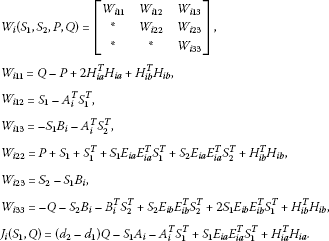Therefore, we finally obtain from (3.9) and the condition (ii) that

$\mathrm{\Delta }V\left(k\right)<{x}^{T}\left(k\right){J}_{i}\left({S}_{1},Q\right)x\left(k\right),\phantom{\rule{1em}{0ex}}\mathrm{\forall }i=1,2,\dots ,N,k=0,1,2,\dots .$

We now apply the condition (i) and Proposition 2.1, the system ${J}_{i}\left({S}_{1},Q\right)$ is strictly complete, and the sets ${\alpha }_{i}$ and ${\overline{\alpha }}_{i}$ by (3.1) are well defined such that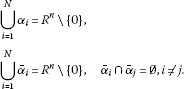Therefore, for any $x\left(k\right)\in {R}^{n}$$k=1,2,\dots$, there exists $i\in \left\{1,2,\dots ,N\right\}$ such that $x\left(k\right)\in {\overline{\alpha }}_{i}$. By choosing switching rule as $\gamma \left(x\left(k\right)\right)=i$ whenever $x\left(k\right)\in {\overline{\alpha }}_{i}$, from the condition (3.9) we have

$\mathrm{\Delta }V\left(k\right)\le {x}^{T}\left(k\right){J}_{i}\left({S}_{1},Q\right)x\left(k\right)<0,\phantom{\rule{1em}{0ex}}k=1,2,\dots ,$

which, combining the condition (3.2) and the Lyapunov stability theorem , concludes the proof of the theorem. □

### 3.2 Stabilization

Consider uncertain control discrete-time systems with interval time-varying delay of the form

$\begin{array}{r}x\left(k+1\right)=\left({A}_{\gamma }+\mathrm{\Delta }{A}_{\gamma }\left(k\right)\right)x\left(k\right)+\left({D}_{\gamma }+\mathrm{\Delta }{D}_{\gamma }\left(k\right)\right)u\left(k\right),\phantom{\rule{1em}{0ex}}k=0,1,2,\dots ,\\ x\left(k\right)={v}_{k},\phantom{\rule{1em}{0ex}}k=-{d}_{2},-{d}_{2}+1,\dots ,0,\end{array}$
(3.10)

where $x\left(k\right)\in {R}^{n}$ is the state, $u\left(k\right)\in {R}^{m}$, $m\le n$, is the control input, $\gamma \left(\cdot \right):{R}^{n}\to \mathcal{N}:=\left\{1,2,\dots ,N\right\}$ is the switching rule, which is a function depending on the state at each time and will be designed. A switching function is a rule which determines a switching sequence for a given switching system. Moreover, $\gamma \left(x\left(k\right)\right)=i$ implies that the system realization is chosen as the i th system, $i=1,2,\dots ,N$. It is seen that the system (2.1) can be viewed as an autonomous switched system in which the effective subsystem changes when the state $x\left(k\right)$ hits predefined boundaries. We consider a delayed feedback control law

$u\left(k\right)=\left({C}_{i}+\mathrm{\Delta }{C}_{i}\left(k\right)\right)x\left(k-d\left(k\right)\right),\phantom{\rule{1em}{0ex}}k=-{h}_{2},\dots ,0,$
(3.11)

and ${C}_{i}$, $i=1,2,\dots ,N$ is the controller gain to be determined. ${A}_{i}$, ${D}_{i}$, $i=1,2,\dots ,N$ are given constant matrices and the time-varying uncertain matrices $\mathrm{\Delta }{A}_{i}\left(k\right)$, $\mathrm{\Delta }{D}_{i}\left(k\right)$, and $\mathrm{\Delta }{C}_{i}\left(k\right)$ are defined by: $\mathrm{\Delta }{A}_{i}\left(k\right)={E}_{ia}{F}_{ia}\left(k\right){H}_{ia},\mathrm{\Delta }{D}_{i}\left(k\right)={E}_{id}{F}_{id}\left(k\right){H}_{id}$, and $\mathrm{\Delta }{C}_{i}\left(k\right)={E}_{ic}{F}_{ic}\left(k\right){H}_{ic}$ where ${E}_{ia}$, ${E}_{id}$, ${E}_{ic}$, ${H}_{ia}$, ${H}_{id}$, ${H}_{ic}$ are known constant real matrices with appropriate dimensions. ${F}_{ia}\left(k\right)$, ${F}_{id}\left(k\right)$, ${F}_{ic}\left(k\right)$ are unknown uncertain matrices satisfying

${F}_{ia}^{T}\left(k\right){F}_{ia}\left(k\right)\le I,\phantom{\rule{2em}{0ex}}{F}_{id}^{T}\left(k\right){F}_{id}\left(k\right)\le I,\phantom{\rule{2em}{0ex}}{F}_{ic}^{T}\left(k\right){F}_{ic}\left(k\right)\le I,\phantom{\rule{1em}{0ex}}k=0,1,2,\dots .$
(3.12)

The time-varying function $d\left(k\right)$ satisfies the following condition:

$0<{d}_{1}\le d\left(k\right)\le {d}_{2},\phantom{\rule{1em}{0ex}}\mathrm{\forall }k=0,1,2,\dots .$

Remark 3.1 It is worth noting that the time delay is a time-varying function belonging to a given interval, in which the lower bound of delay is not restricted to zero.

Applying the feedback controller (3.11) to the system (3.10), the closed-loop discrete time-delay system is

$x\left(k+1\right)=\left({A}_{i}+\mathrm{\Delta }{A}_{i}x\left(k\right)+\left({D}_{i}+\mathrm{\Delta }{D}_{i}\right)\left({C}_{i}+\mathrm{\Delta }{C}_{i}\right)x\left(k-d\left(k\right)\right),\phantom{\rule{1em}{0ex}}k=0,1,2,\dots .$
(3.13)

Definition 3.1 The uncertain switched control system (3.10) is robustly stabilizable if there is a delayed feedback control (3.11) such that the switched system (3.13) is robustly stable.

Let us set

${W}_{i}=\left[\begin{array}{ccc}{W}_{i11}& {W}_{i12}& {W}_{i13}\\ \ast & {W}_{i22}& {W}_{i23}\\ \ast & \ast & {W}_{i33}\end{array}\right],$

where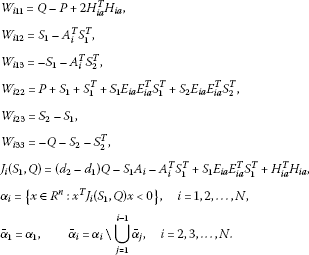(3.14)

Theorem 3.2 The switched control system (3.10) is robustly stabilizable by the delayed feedback control (3.11), where

$\left({C}_{i}+\mathrm{\Delta }{C}_{i}\right)={\left({D}_{i}+\mathrm{\Delta }{D}_{i}\right)}^{T}{\left[\left({D}_{i}+\mathrm{\Delta }{D}_{i}\right){\left({D}_{i}+\mathrm{\Delta }{D}_{i}\right)}^{T}\right]}^{-1},\phantom{\rule{1em}{0ex}}i=1,2,\dots ,N,$

if there exist symmetric matrices$P>0$, $Q>0$and matrices${S}_{1}$, ${S}_{2}$satisfying the following conditions

1. (i)

$\mathrm{\exists }{\delta }_{i}\ge 0$, $i=1,2,\dots ,N$, ${\sum }_{i=1}^{N}{\delta }_{i}>0:{\sum }_{i=1}^{N}{\delta }_{i}{J}_{i}\left({S}_{1},Q\right)<0$,

2. (ii)

${W}_{i}\left({S}_{1},{S}_{2},P,Q\right)<0$, $i=1,2,\dots ,N$.

The switching rule is chosen as$\gamma \left(x\left(k\right)\right)=i$, whenever$x\left(k\right)\in {\overline{\alpha }}_{i}$.

Proof Using the feedback control (3.11), the closed-loop system leads to the system (2.1), where

$\begin{array}{rcl}\left({B}_{i}+\mathrm{\Delta }{B}_{i}\right)& =& \left({D}_{i}+\mathrm{\Delta }{D}_{i}\right)\left({C}_{i}+\mathrm{\Delta }{C}_{i}\right)\\ =& \left({D}_{i}+\mathrm{\Delta }{D}_{i}\right){\left({D}_{i}+\mathrm{\Delta }{D}_{i}\right)}^{T}{\left[\left({D}_{i}+\mathrm{\Delta }{D}_{i}\right){\left({D}_{i}+\mathrm{\Delta }{D}_{i}\right)}^{T}\right]}^{-1}=I.\end{array}$

Since ${S}_{1}\left({B}_{i}+\mathrm{\Delta }{B}_{i}\right)={S}_{1}$, ${\left({B}_{i}+\mathrm{\Delta }{B}_{i}\right)}^{T}{S}_{1}^{T}={S}_{1}^{T}$, ${S}_{2}\left({B}_{i}+\mathrm{\Delta }{B}_{i}\right)={S}_{2}$, ${\left({B}_{i}+\mathrm{\Delta }{B}_{i}\right)}^{T}{S}_{2}^{T}={S}_{2}^{T}$, the stability condition of the closed-loop system (3.13), by Theorem 3.1, is immediately derived. □

Remark 3.2 Note that the results proposed in  for switching systems to be asymptotically stable under an arbitrary switching rule. The asymptotic stability for switching linear discrete-time delay systems studied in  was limited to constant delays. In , a class of switching signals has been identified for the considered switched discrete-time delay systems to be stable under the averaged well time scheme.

## 4 Numerical examples

Example 4.1 (Stability)

Consider the uncertain switched discrete-time system (2.1), where the delay function $d\left(k\right)$ is given by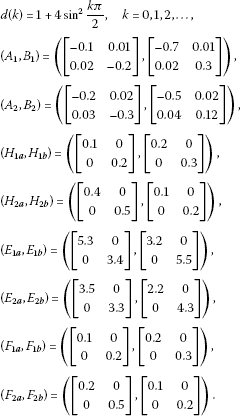By LMI toolbox of Matlab, we find that the conditions (i), (ii) of Theorem 3.1 are satisfied with ${d}_{1}=1$, ${d}_{2}=5$, ${\delta }_{1}=1$, ${\delta }_{2}=1$, and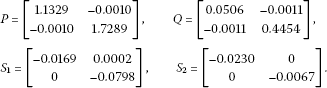In this case, we have

$\left({J}_{1}\left({S}_{1},Q\right),{J}_{2}\left({S}_{1},Q\right)\right)=\left(\begin{array}{c}\left[\begin{array}{cc}-0.2170& -0.0026\\ -0.0026& -1.8633\end{array}\right],\left[\begin{array}{cc}-0.3591& -0.0016\\ -0.0016& -2.0531\end{array}\right]\end{array}\right).$

Moreover, the sum

${\delta }_{1}{J}_{1}\left(R,Q\right)+{\delta }_{2}{J}_{2}\left(R,Q\right)=\left[\begin{array}{cc}-0.5761& -0.0042\\ -0.0042& -3.9164\end{array}\right],$

is negative definite, i.e., the first entry in the first row and the first column $-0.5761<0$ is negative and the determinant of the matrix is positive. The sets ${\alpha }_{1}$ and ${\alpha }_{2}$ are given as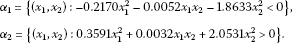Obviously, the union of these sets is equal to ${R}^{2}\setminus \left\{0\right\}$. The switching regions are defined as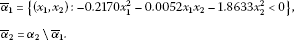By Theorem 3.1 the uncertain system is robustly stable and the switching rule is chosen as $\gamma \left(x\left(k\right)\right)=i$ whenever $x\left(k\right)\in {\overline{\alpha }}_{i}$.

Example 4.2 (Stabilization)

Consider the uncertain switched discrete-time control system (3.10), where the delay function $d\left(k\right)$ is given by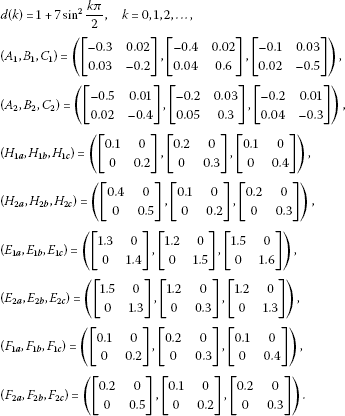By LMI toolbox of Matlab, we find that the conditions (i), (ii) of Theorem 3.2 are satisfied with ${d}_{1}=1$, ${d}_{2}=8$, ${\delta }_{1}=2$, ${\delta }_{2}=1$, and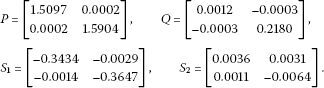In this case, we have

$\left({J}_{1}\left({S}_{1},Q\right),{J}_{2}\left({S}_{1},Q\right)\right)=\left(\begin{array}{c}\left[\begin{array}{cc}-1.5519& -0.0176\\ -0.0176& -1.6807\end{array}\right],\left[\begin{array}{cc}-1.6306& -0.0097\\ -0.0097& -1.7089\end{array}\right]\end{array}\right).$

Moreover, the sum

${\delta }_{1}{J}_{1}\left(R,Q\right)+{\delta }_{2}{J}_{2}\left(R,Q\right)=\left[\begin{array}{cc}-4.7344& -0.0449\\ -0.0449& -5.0703\end{array}\right],$

is negative definite, i.e., the first entry in the first row and the first column $-4.7344<0$ is negative and the determinant of the matrix is positive. The sets ${\alpha }_{1}$ and ${\alpha }_{2}$ are given as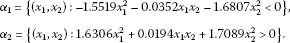Obviously, the union of these sets is equal to ${R}^{2}\setminus \left\{0\right\}$. The switching regions are defined as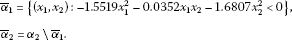By Theorem 3.2, the control system is robustly stabilizable and the switching rule is $\sigma \left(x\left(k\right)\right)=i$ whenever $x\left(k\right)\in {\overline{\mathrm{\Omega }}}_{i}$, the delayed feedback control is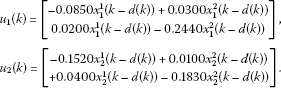## 5 Conclusion

This paper has proposed a switching design for the robust stability and stabilization of uncertain switched linear discrete-time systems with interval time-varying delays. Based on the discrete Lyapunov functional, a switching rule for the robust stability and stabilization for the uncertain system is designed via linear matrix inequalities.

## References

1. Liberzon D, Morse AS: Basic problems in stability and design of switched systems. IEEE Control Syst. Mag. 1999, 19: 57–70.

2. Dong Y, Liu J, Mei S, Li M: Stabilization for switched nonlinear time-delay systems. Nonlinear Anal. Hybrid Syst. 2011, 5: 78–88. 10.1016/j.nahs.2010.09.001

3. Wang R, Liu X, Guan Z: Robustness and stability analysis for a class of nonlinear switched systems with impulse effects. Dyn. Syst. Appl. 2004, 14: 233–248.

4. Peleties P, Decarlo R: Asymptotic stability of m-switched systems using Lyapunov-like functions. Proceedings of the 1991 American Control Conference 1991, 1679–1684.

5. Ishii H, Francis BA: Stabilizing a linear system by switching control with dwell time. IEEE Trans. Autom. Control 2002, 47: 1962–1973. 10.1109/TAC.2002.805689

6. Branicky MS: Multiple Lyapunov functions and other analysis tools for switched and hybrid systems. IEEE Trans. Autom. Control 1998, 43: 475–482. 10.1109/9.664150

7. Skafidas E, Evans RJ, Savkin AV, Petersen IR: Stability results for switched controller systems. Automatica 1999, 35: 553–564. 10.1016/S0005-1098(98)00167-8

8. Jianyin F, Kai L: Stabilization of nonlinear systems under arbitrary switching law. Proceedings of the 30th Chinese Control Conference 2010, 641–643.

9. Dong Y, Fan J, Mei S: Quadratic stabilization of switched nonlinear systems. Sci. China Ser. F 2009, 52: 999–1006. 10.1007/s11432-009-0111-z

10. Ferrari-Trecate G, Cuzzola FA, Mignone D, Morari M: Analysis of discrete-time piecewise affine and hybrid systems. Automatica 2002, 38: 2138–2146.

11. Daafouz J, Riedinger P, Iung C: Stability analysis and control synthesis for switched systems: a switched Lyapunov function approach. IEEE Trans. Autom. Control 2002, 47: 1883–1887. 10.1109/TAC.2002.804474

12. Phat VN: Robust stability and stabilizability of uncertain linear hybrid systems with state delays. IEEE Trans. Circuits Syst. II, Express Briefs 2005, 52: 94–98.

13. Sun YG, Wang L, Xie G:Delay-dependent robust stability and ${H}_{\mathrm{\infty }}$ control for uncertain discrete-time switched systems with mode-dependent time delays. Appl. Math. Comput. 2007, 187: 1228–1237. 10.1016/j.amc.2006.09.053

14. Zhang L, Shi P, Wang C, Gao H:Robust ${H}_{\mathrm{\infty }}$ filtering for switched linear discrete-time systems with polytopic uncertainties. Int. J. Adapt. Control Signal Process. 2006, 20: 291–304. 10.1002/acs.901

15. Ji Z, Wang L: Quadratic stabilization of uncertain discrete-time switched linear systems. Proceeding of the IEEE International Conference on Systems, Man and Cybernetics (SMC ’04) 2004, 4555–4560.

16. Zhai G, Lin H, Michel AN, Yasuda K: Stability analysis for switched systems with continuous-time and discrete-time subsystems. Proceedings of the American Control Conference (AAC ’04) 2004, 4555–4560.

17. Sun YG, Wang L: Stabilization of planar discrete-time switched systems: switched Lyapunov functional approach. Nonlinear Anal. Hybrid Syst. 2008, 2: 1062–1068. 10.1016/j.nahs.2008.09.004

18. Chiou JS, Cheng CM: Stabilization analysis for a class of switched discrete-time systems. Proceeding of the IEEE International Conference on Systems, Man and Cybernetics (SMC ’06) 2006, 4535–4540.

19. da la Sen M: Quadratic stability and stabilization of switched dynamic systems with uncommensurate internal point delays. Appl. Math. Comput. 2007, 185: 508–526. 10.1016/j.amc.2006.07.048

20. Liu XZ, Yuan SA:On designing ${H}_{\mathrm{\infty }}$ fault estimator for switched nonlinear systems of neutral type. Commun. Nonlinear Sci. Numer. Simul. 2011, 16: 4379–4389. 10.1016/j.cnsns.2011.03.017

21. Xiang ZR, Sun Y-N, Chen QW: Robust reliable stabilization of uncertain switched neutral systems with delayed switching. Appl. Math. Comput. 2011, 217: 9835–9844. 10.1016/j.amc.2011.04.082

22. Zhang W, Abate A, Hu J, Vitus MP: Exponential stabilization of discrete-time switched linear systems. Automatica 2009, 45: 2526–2536. 10.1016/j.automatica.2009.07.018

23. Sun YG, Wang L, Xie G: Delay-dependent robust stability and stabilization for discrete-time switched systems with mode-dependent time-varying delays. Appl. Math. Comput. 2006, 180: 428–435. 10.1016/j.amc.2005.12.028

24. Phat VN, Botmart T, Niamsup P: Switching design for exponential stability of a class of nonlinear hybrid time-delay systems. Nonlinear Anal. Hybrid Syst. 2009, 3: 1–10. 10.1016/j.nahs.2008.10.001

25. Mahmoud MS: Switched Time-Delay Systems: Stability and Control. Springer, Boston; 2010.

26. Mahmoud MS, Nounou MN, Nounou HN: Analysis and synthesis of uncertain switched discrete-time systems. IMA J. Math. Control Inf. 2007, 24: 245–257.

27. Mahmoud MS:Delay-dependent ${H}_{2}$ filtering of a class of switched discrete-time state-delay systems. J. Signal Process. 2008, 88(11):2709–2719. 10.1016/j.sigpro.2008.05.014

28. Mahmoud MS:Switched discrete-time systems with time-varying delays: a generalized ${H}_{2}$ approach. Comput. Math. Appl. 2009, 57(1):79–95. 10.1016/j.camwa.2008.10.066

29. Mahmoud MS:Generalized ${H}_{2}$ control of switched discrete-time systems with unknown delays. Appl. Math. Comput. 2009, 311(1):33–44.

30. Mahmoud MS, Saif AW, Shi P:Stabilization of linear switched delay systems: ${H}_{2}$ and ${H}_{\mathrm{\infty }}$ methods. J. Optim. Theory Appl. 2009, 142(3):583–601. 10.1007/s10957-009-9527-2

31. Mahmoud MS, AL-Sunni FM, Shi Y: Switched discrete-time delay systems: delay-dependent analysis and synthesis. Circuits Syst. Signal Process. 2009, 28(5):735–761. 10.1007/s00034-009-9107-6

32. Li X, Gao H: A new model transformation of discrete-time systems with time-varying delay and its application to stability analysis. IEEE Trans. Autom. Control 2011, 56: 2172–2178.

33. Gao H, Li X:${H}_{\mathrm{\infty }}$ filtering for discrete-time state-delayed systems with finite frequency specifications. IEEE Trans. Autom. Control 2011, 56: 2935–2941.

34. Ratchagit K: Asymptotic stability of nonlinear delay-difference system via matrix inequalities and application. Int. J. Comput. Methods 2009, 6: 389–397. 10.1142/S0219876209001899

35. Phat VN, Kongtham Y, Ratchagit K: LMI approach to exponential stability of linear systems with interval time-varying delays. Linear Algebra Appl. 2012, 436: 243–251. 10.1016/j.laa.2011.07.016

36. Phat VN, Ratchagit K: Stability and stabilization of switched linear discrete-time systems with interval time-varying delay. Nonlinear Anal. Hybrid Syst. 2011, 5: 605–612. 10.1016/j.nahs.2011.05.006

37. Ratchagit K, Phat VN: Stability criterion for discrete-time systems. J. Inequal. Appl. 2010., 2010:

38. Uhlig F: A recurring theorem about pairs of quadratic forms and extensions. Linear Algebra Appl. 1979, 25: 219–237.

39. Agarwal RP: Difference Equations and Inequalities. Dekker, New York; 2000.

40. Lien CH, Yu KW, Chung YJ, Lin YF, Chung LY, Chen JD: Exponential stability analysis for uncertain switched neutral systems with interval-time-varying state delay. Nonlinear Anal. Hybrid Syst. 2009, 3: 334–342. 10.1016/j.nahs.2009.02.010

41. Xie G, Wang L: Quadratic stability and stabilization of discrete-time switched systems with state delay. Proc. of the IEEE Conference on Decision and Control, Atlantics 2004, 3235–3240.

42. Zhai GS, Hu B, Yasuda K, Michel A: Qualitative analysis of discrete- time switched systems. Proc. of the American Control Conference 2002, 1880–1885.

43. Zhang WA, Yu L: Stability analysis for discrete-time switched time-delay systems. Automatica 2009, 45: 2265–2271. 10.1016/j.automatica.2009.05.027

## Acknowledgements

This work is partially supported by the Thai Research Fund Grant, the Higher Education Commission and Faculty of Science, Maejo University, Thailand (for the first and third authors) and also by Centre of Excellence in Mathematics, the Commission on Higher Education, Thailand (for the second author). The authors thank anonymous reviewers for valuable comments and suggestions, which allowed us to improve the paper.

## Author information

Authors

### Corresponding author

Correspondence to G Rajchakit.

### Competing interests

The authors declare that they have no competing interests.

### Authors’ contributions

The authors contributed equally and significantly in writing this paper. The authors read and approved the final manuscript.

## Rights and permissions

Open Access This article is distributed under the terms of the Creative Commons Attribution 2.0 International License (https://creativecommons.org/licenses/by/2.0), which permits unrestricted use, distribution, and reproduction in any medium, provided the original work is properly cited.

Reprints and Permissions

Rajchakit, G., Rojsiraphisal, T. & Rajchakit, M. Robust stability and stabilization of uncertain switched discrete-time systems. Adv Differ Equ 2012, 134 (2012). https://doi.org/10.1186/1687-1847-2012-134

• Accepted:

• Published:

• DOI: https://doi.org/10.1186/1687-1847-2012-134

### Keywords

• switching design
• uncertain discrete system
• robust stability and stabilization
• Lyapunov function
• linear matrix inequality